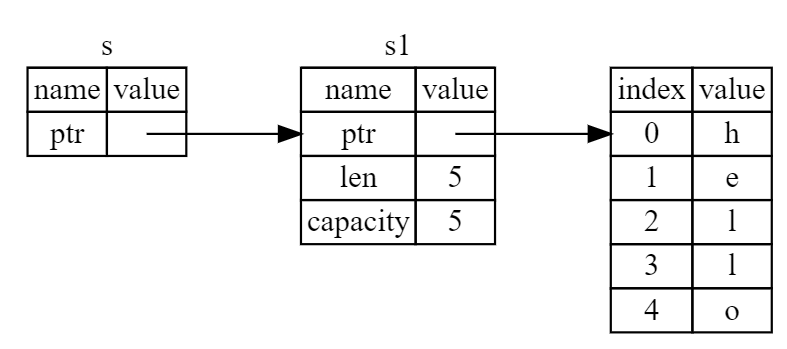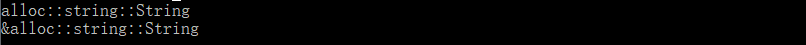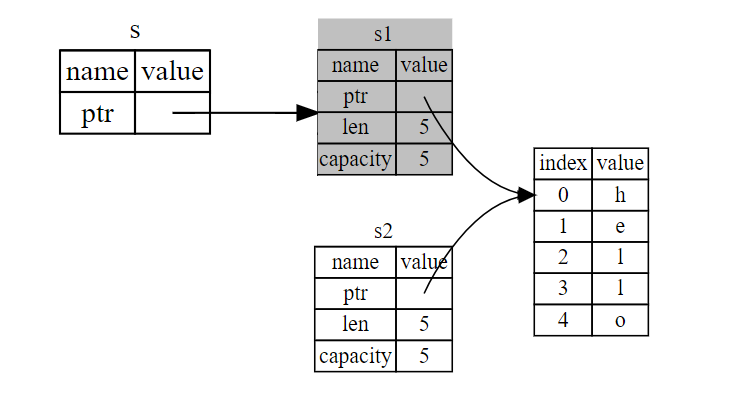# 悬挂引用是如何被 Rust 消灭的？Rust 承诺：引用始终有效

## 一 引用的内存模型

fn print_type_of<T>(_: &T) {    println!("{}", std::any::type_name::<T>())}fn main() {    let s1 = String::from("hello");    let s = &s1;        print_type_of(& s1);	print_type_of(& s);}s 和 s1，是两种不同的类型，可以用下面的代码把类型打印来看。之所以 s 和 s1 用起来没差别，是因为引用 s 能自动解引用。

fn print_type_of<T>(_: &T) { println!("{}", std::any::type_name::<T>())}​fn main() { let s1 = String::from("hello"); let s = &s1; print_type_of(& s1); print_type_of(& s);}## 二 悬挂引用问题

• 空指针（null pointer）：指针值为 Null；

• 野指针（wild pointer）：未经初始化的“垃圾值”地址；

• 悬挂指针（dangling pointer）：指向已经释放的地址；Rust 必须在编译期就能检查出来引用的有效性

## 三 策略 1：借用检查器检查引用的生命周期

{    let r;                // ---------+-- 'a                          //          |    {                     //          |        let x = 5;        // -+-- 'b  |        r = &x;           //  |       |    }                     // -+       |                          //          |    println!("r: {}", r); //          |}                         // ---------+

Rust 会检查所有的可能性，包括控制条件里的所有可能路径。下面代码在编译时依然会得到error[E0597]: 'x' does not live long enough

{    	let y = 6;              // --------------------+-- 'a    let r;                  // ------+-- 'b        |    {                       //       |             |             let x = 5;          // ------|-------+--'c |              if x > y{           //       |       |     |            r = &x;         //       |       |     |        }                   //       |       |     |        else{               //       |       |     |            r = &y;         //       |       |     |        }                   //       |       |     |    }                       // ------|-------+     |    println!("r: {}", r);   //       |             |                            // ------+             |}                           // --------------------+

{    let mut x = String::from("Hello");      // ----------------+-- 'a    x.push_str(", world");				    //                 |    let r1 = &x;                            // -----'b	       |	         let r2 = &mut x;                        // -----'c		   |    let r3 = &mut x;                        // ---+-'d         |    r3.push_str("!");                       //    |            |    println!("r3: {}", r3);                 //    |            |                                                                     // ---+            | }                                           // ----------------+

## 四 策略 2：函数定义中，不能返回所有权属于函数的引用

fn test(r:&i32)-> &i32{    let x = 5;        println!("r is: {}", r);    &x}

## 五 策略 3：函数签名生命周期标注

### 编译器的局限

{    let y = 6;                  //----------------------+--'a    let r1;                     //--------+--'c         |    let r2;                     //--------|------+--'b  | 	{                           //        |      |      |        let x = 5;              //--+--'d |      |      |        r1 = bigger(&x, &y);    //  |     |      |      |        r2 = second(&x, &y);    //  |     |      |      |        println!("{}", r1);     //  |     |      |      |     }                           //--+     |      |      |    println!("{}", r2);         //--------+      |      |                                //---------------+      |}                               //----------------------+ 

• r1的生命周期'c是和x的生命周期'd比呢？还是和y的生命周期'a比？

• r2的生命周期'b是和x的生命周期'd比呢？还是和y的生命周期'a比？

fn bigger(s: &i32, t: &i32) -> &i32{    if s > t{        s    }    else{        t    }}fn second(s: & i32, t: & i32) -> &i32{    t}

### 输入和输出生命周期标注

fn bigger<'a>(s: &'a i32, t: &'a i32) -> &'a i32{    if s > t{        s    }    else{        t    }}fn second<'a>(s: & i32, t: &'a i32) -> &'a i32{    t}

### 标注规则

• 只需在函数签名上进行标注；

• **生命周期用'开头，后面跟一个全小写字符，比如'a；**

• **用尖括号在函数名与参数列表之间声明泛型生命周期参数，例如<'a>；**

• **标注'a并不是一段具体的存活时长，只要满足约束关系即可；**

• **泛型'a会被具体化为 x 与 y 两者中生命周期较短的那一个；**

### 函数实现与签名标注的兼容

fn second<'a>(s: &'a i32, t: & i32) -> &'a i32{    t}

• 函数实现处，必须兼容签名，由 Rust 编译器进行检查；

• 函数调用处，必须遵守签名，由 Rust 编译器进行检查；

## 七 相关文章### 袁承兴

Rust语言爱好者。 2018.05.01 加入

## 评论